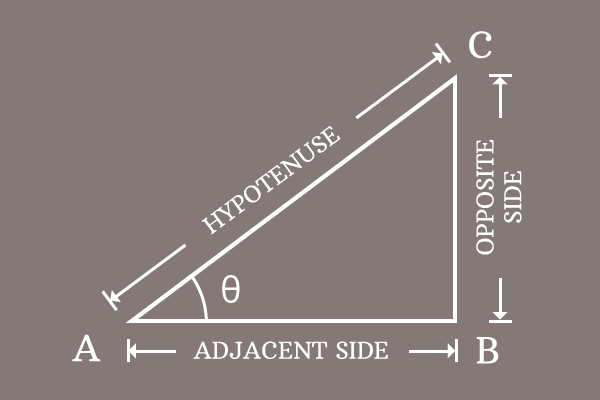# Pythagorean identity of Cosecant and Cotangent functions

The subtraction of square of cotangent function from cosecant function at an angle equals to one, is called Pythagorean identity for cosecant and cotangent functions.

## Formula

$\csc^2{\theta} \,-\, \cot^2{\theta} = 1$

### Proof$\overline{BC}$, $\overline{AB}$ and $\overline{AC}$ are the opposite side, adjacent side and hypotenuse of the $\Delta CAB$ respectively and the associated lengths are $BC$, $AB$ and $AC$.

#### Expressing Relation between sides

Write the mathematical relation between three sides of the right angled triangle as per Pythagorean Theorem.

${AC}^2 = {BC}^2 + {AB}^2$

$\implies {BC}^2 + {AB}^2 = {AC}^2$

#### Adjusting the mathematical relation

Do an adjustment acceptably to express the equation in terms of cosecant and cotangent functions. It can be done by dividing both sides of the equation by the square of length of the side $\overline{BC}$.

$\implies \dfrac{{BC}^2 + {AB}^2}{{BC}^2} = \dfrac{{AC}^2}{{BC}^2}$

$\implies \dfrac{{BC}^2}{{BC}^2} + \dfrac{{AB}^2}{{BC}^2} = \dfrac{{AC}^2}{{BC}^2}$

$\implies \Bigg(\dfrac{BC}{BC}\Bigg)^2 + \Bigg(\dfrac{AB}{BC}\Bigg)^2 = \Bigg(\dfrac{AC}{BC}\Bigg)^2$

$\implies 1 + \Bigg(\dfrac{AB}{BC}\Bigg)^2 = \Bigg(\dfrac{AC}{BC}\Bigg)^2$

#### Expressing the Equation in terms of Co-secant and Co-tangent

Now, express the ratios of sides in terms of trigonometric functions according to the $\Delta BAC$.

$\dfrac{AB}{BC} = \cot{\theta}$

$\dfrac{AC}{BC} = \csc{\theta}$

Substitute the ratios of the sides by the associated trigonometric functions.

$\implies 1 + (\cot{\theta})^2 = (\csc{\theta})^2$

$\implies 1 + \cot^2{\theta} = \csc^2{\theta}$

$\implies 1 = \csc^2{\theta} \,-\, \cot^2{\theta}$

$\,\,\, \therefore \,\,\,\,\,\, \csc^2{\theta} \,-\, \cot^2{\theta} = 1$

Therefore, it is proved that the subtraction of square of cotangent function from square of cosecant function at an angle is equal to one. This trigonometric identity is derived according to the Pythagoras Theorem. Hence, it is generally known as the Pythagorean identity for cosecant and cotangent functions.

Latest Math Topics
Email subscription
Math Doubts is a best place to learn mathematics and from basics to advanced scientific level for students, teachers and researchers. Know more
Follow us on Social Media
###### Math Problems

Learn how to solve easy to difficult mathematics problems of all topics in various methods with step by step process and also maths questions for practising.